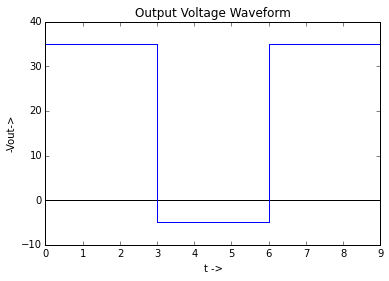# Chapter 5 , Diode Applications - DC Power Supplies and Waveshaping Circuits¶

## Example 5.1 , Page Number 140¶

In :
import math

#Variables

VSrms = 10                #Supply voltage
VSmax = 10* 2**0.5        #Peak value of supply voltage (in volts)
RF = 0.3                  #Forward resistance (in ohm)
RL = 2                    #Load resistance (in ohm)

#Calculation

Imax = VSmax/(RL + RF)    #Peak value of current in load (in Ampere)
Idc = Imax/math.pi        #DC ouput current (in Ampere)
Irms = Imax/2             #RMS value of output current (in Ampere)

#Result

print "Idc = ",round(Idc,3)," A."
print "Irms = ",round(Irms,3)," A."

#Slight variation due to higher precision.

Idc =  1.957  A.
Irms =  3.074  A.


## Example 5.2 , Page Number 141¶

In :
import math

#Variables

VSrms = 220.0                   #Supply voltage
VSmax = 220.0 * 2**0.5          #Peak value of supply voltage (in volts)
RF = 100.0                      #Forward resistance (in ohm)
RL = 2.0 * 10**3                #Load resistance (in ohm)

#Calculation

Imax = VSmax/(RL + RF)          #Maximum value of current (in Ampere)
Idc = Imax/math.pi              #DC ouput current (in Ampere)
Irms = Imax/2                   #RMS value of output current (in Ampere)
PIV = VSmax                     #Peak inverse voltage (in volts)
Vdc = Idc*RL                    #Load output voltage (in volts)
Pdc = Idc**2 * RL               #DC output power (in watt)
Pac = Imax**2/4*(RF + RL)       #AC input power (in watt)
gamma = ((Irms/Idc)**2 - 1)**.5 #Ripple factor
TUF = 0.286/(1 + RF/RL)         #Transformer utilisation factor
eeta = Pdc/Pac * 100            #Rectification efficiency

#Result

print "Imax = ",round(Imax * 10**3,3)," mA.\nIdc = ",round(Idc * 10**3,2)," mA.\nIrms = ",round(Irms * 10**3,3)," mA."
print "PIV = ",round(PIV,3)," V."
print "Load output voltage = ",round(Vdc,2)," V."
print "DC output power = ",round(Pdc,3)," W.\nAC input power = ",round(Pac,3)," W."
print "Ripple factor = ",round(gamma,2),"."
print "Transformer utilisation factor = ",round(TUF,4),"."
print "Rectification efficiency = ",round(eeta,1),"%."

Imax =  148.156  mA.
Idc =  47.16  mA.
Irms =  74.078  mA.
PIV =  311.127  V.
Load output voltage =  94.32  V.
DC output power =  4.448  W.
AC input power =  11.524  W.
Ripple factor =  1.21 .
Transformer utilisation factor =  0.2724 .
Rectification efficiency =  38.6 %.


## Example 5.3 , Page Number 141¶

In :
#Variables

VNL = 44.0             #No load voltage (in volts)
VFL = 42.0             #Full load voltage (in volts)

#Calculation

Reg = (VNL - VFL)/VFL * 100        #Percentage voltage regulation

#Result

print "Percentage voltage regulation : ",round(Reg,2)," %."

Percentage voltage regulation :  4.76  %.


## Example 5.4 , Page Number 141¶

In :
import math

#Variables

RF = 10                  #Forward resistance (in ohm)
IL = 100 * 10**-3        #Load current (in Ampere)
VSrms = 12               #RMS value of supply voltage (in volts)
VSmax = 12 * 2**0.5      #Maximum value of supply voltage (in volts)
Idc = 0.1                #DC current (in Ampere)

#Calculation

Vdc = VSmax/math.pi - Idc*RF    #DC output voltage (in volts)
PIV = VSmax                     #Peak inverse voltage (in volts)

#Result

print "DC output voltage : ",round(Vdc,1)," V."
print "PIV : ",round(PIV)," V."

DC output voltage :  4.4  V.
PIV :  17.0  V.


## Example 5.5 , Page Number 146¶

In :
import math

#Variables

VSmax = 60.0                           #Maximum value of supply voltage (in volts)
RF = 50.0                              #Forward resistance (in ohm)
RL = 500.0                             #Load resistance (in ohm)

#Calculation

Imax = VSmax/(RL + RF)                #Peak current (in Ampere)
Idc = 2*Imax/math.pi                  #Average current (in Ampere)
Irms = Imax/2**0.5                    #RMS value of current (in Ampere)
r = ((Irms/Idc)**2 - 1)**0.5          #Ripple factor
n = 0.812/(1 + RF/RL)*100             #Efficiency of rectifier

#Result

print "Peak value of current : ",round(Imax,3)," A.\nAverage value of current : ",round(Idc,4)," A.\nRMS value of current : ",round(Irms,3)," A."
print "Ripple factor : ",round(r,3),"."
print "Efficiency of rectifier : ",round(n,2),"%."

Peak value of current :  0.109  A.
Average value of current :  0.0694  A.
RMS value of current :  0.077  A.
Ripple factor :  0.483 .
Efficiency of rectifier :  73.82 %.


## Example 5.6 , Page Number 147¶

In :
import math

#Variables

VSmax = 12 * 2**0.5            #Peak value of supply voltage (in volts)
RL = 100.0                     #Load resistance (in ohm)

#Calculation

Idc = 2*VSmax/(RL*math.pi)     #Average current (in Ampere)
Vdc = Idc * RL                 #Average voltage (in volts)
PIV = 2*VSmax                  #Peak inverse voltage (in volts)

#Result

print "DC output voltage : ",round(Vdc,1)," V."
print "DC load current : ",round(Idc * 10**3)," mA."
print "PIV rating required : ",round(PIV,2)," V."

DC output voltage :  10.8  V.
DC load current :  108.0  mA.
PIV rating required :  33.94  V.


## Example 5.7 , Page Number 153¶

In :
import math

#Variables

VLmax = VSmax = 40.0         #Peak value of supply voltage (in volts)
f = 50                       #Frequency (in Hertz)
w = 2*math.pi*50             #Angular frequency (in rad/sec)
L = 2.0                      #Inductance (in Henry)
C = 40 * 10**-6              #Capacitance (in Farad)

#Calculation

Vdc = 2*VSmax/math.pi        #Average voltage (in bolts)
r = 1/(6*2**0.5*w**2*L*C)    #Ripple factor

#Result

print "Output DC voltage : ",round(Vdc,2),"V."
print "Ripple fator : ",round(r,4),"."

Output DC voltage :  25.46 V.
Ripple fator :  0.0149 .


## Example 5.8 , Page Number 161¶

In :
#Variables

Vo = 0.7                    #Barrier potential (in volts)
Vinpeak = 15                #Peak input voltage (in volts)

#Calculation

Voutpeak = Vinpeak - Vo     #Peak output voltage (in volts)

#Result

print "Peak output voltage : ",Voutpeak," V."

Peak output voltage :  14.3  V.


## Example 5.9 , Page Number 161¶

In :
import math

#Variables

R1 = 2.0 * 10**3                #Resistance1 (in ohm)
R2 = 1.0 * 10**3                #Resistance2 (in ohm)
Vinpeak = 10                    #peak input voltage (in volts)

#Calculation

RL = R1*R2/(R1+R2)              #Load resistance (in ohm)
Voutpeak = Vinpeak*RL/(R2+RL)   #Peak voltage across load resistance (in ohm)
Vrms = Voutpeak/math.pi         #RMS value of output voltage (in volts)

#Result

print "Output voltage (rms value) : ",round(Vrms,2)," V."

Output voltage (rms value) :  1.27  V.


## Example 5.10 , Page Number 162¶

In :
import math
import numpy
%matplotlib inline
from matplotlib.pyplot import plot,title,xlabel,ylabel,ylim,xlim

#Variables

R1 = 20.0 * 10**3                #Resistance1 (in ohm)
R2 = 10.0 * 10**3                #Resistance2 (in ohm)
Vinpeak = 20                     #peak input voltage (in volts)

#Calculation

RL = R1*R2/(R1+R2)              #Load resistance (in ohm)
Voutpeak = Vinpeak*RL/(R2+RL)   #Peak voltage across load resistance (in ohm)

#Result

print "Voutpeak : ",Voutpeak,"V."

#Graph

x = numpy.linspace(0,math.pi,100)
y = numpy.sin(x)
plot(x,8*y,'b')
ylim(0,9)
xlim(0,math.pi)
title("Output Waveform")
xlabel("t ->")
ylabel("-Vout->")

Voutpeak :  8.0 V.

Out:
<matplotlib.text.Text at 0x6186270>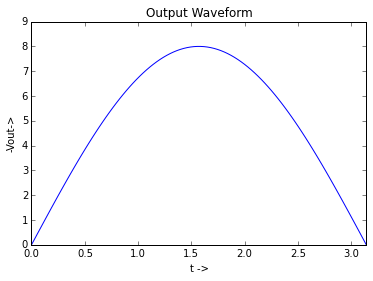## Example 5.11 , Page Number 162¶

In :
import math
import numpy
%matplotlib inline
from matplotlib.pyplot import plot,title,xlabel,ylabel,ylim,xlim

#Variables

Vinpeak = 12.0               #Peak input voltage (in volts)
Vo = 0.7                     #Barrier potential (in volts)
RS = 500                     #Series resistance (in ohm)
RL = 2.5 * 10**3             #Load resistance (in ohm)

#Calculation

Voutpeak = Vinpeak*RL/(RS+RL) #Peak output voltage (in volts)

#Result

print "Peak output voltage : ",Voutpeak," V."

#Graph

x = numpy.linspace(0,math.pi,100)
x1 = numpy.linspace(math.pi,2*math.pi,100)
x2 = numpy.linspace(math.pi,2*math.pi,100)
x3=numpy.linspace(0,8,100)
y2 = numpy.sin(x2)
y = numpy.sin(x)
plot(x,8*y,'b')
plot(x1,-0.7+x1-x1,'b')
plot(x2,12*y2,'--')
plot(x3,0+x3-x3,'k')
ylim(-13,9)
xlim(0,2*math.pi+1)
title("Output Waveform")
xlabel("t ->")
ylabel("-Vout->")

Peak output voltage :  10.0  V.

Out:
<matplotlib.text.Text at 0x6dc1210>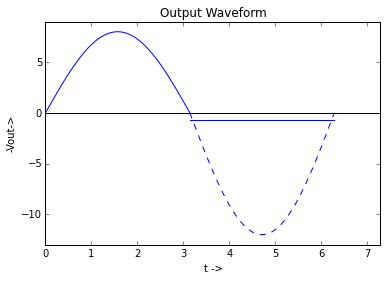## Example 5.12 , Page Number 163¶

In :
import math
import numpy
%matplotlib inline
from matplotlib.pyplot import plot,title,xlabel,ylabel,ylim,xlim

#Variables

Vi = 10                    #Input voltage (in volts)

#Calculation

Vo = Vi * 1.0/2            #Output voltage (in volts)
Vdc = 0.636 * Vo           #DC output voltage (in volts)
PIV = 5                    #Peak inverse voltage (in volts)

#Result

print "PIV of diode : ",PIV,"V."

#Graph

x = numpy.linspace(0,math.pi,100)
y = numpy.sin(x)
x1 = numpy.linspace(math.pi,2*math.pi,100)
y1 = numpy.sin(x1)
ylim(0,8)
xlim(0,2*math.pi)
plot(x,5*(y),'b')
plot(x1,-5*(y1),'b')
plot(x,0+x-x,'k')
plot(x1,0+x1-x1,'k')
plot(x,5+x-x,'--',color='g')
plot(x1,5+x1-x1,'--',color='g')
title("Output Waveform")
xlabel("t ->")
ylabel("-Vout->")

PIV of diode :  5 V.

Out:
<matplotlib.text.Text at 0x7d41190>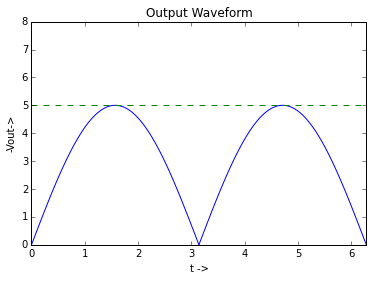## Example 5.13 , Page Number 164¶

In :
import math
import numpy
%matplotlib inline
from matplotlib.pyplot import plot,title,xlabel,ylabel,ylim,xlim,annotate

#Variables

Vimax = 200            #Peak input voltage (in volts)
R = 10 * 10**3         #Resistance (in ohm)
RL = 4 * 10**3         #Load resistance (in ohm)

#Calculation

Iimax = Vimax/R        #Peak input current (in Ampere)
VLmax = Iimax * RL     #Peak voltage across load (in volts)
PIV = 200              #Peak inverse voltage (in volts)

#Result

print "PIV rating of diode :",PIV,"V."

#Graph

x1 = numpy.linspace(0,math.pi,100)
x2 = numpy.linspace(math.pi,2*math.pi,100)
x3 = numpy.linspace(2*math.pi,3*math.pi,100)
y1 = numpy.sin(x1)
y2 = numpy.sin(x2)
y3 = numpy.sin(x3)
plot(x1,80*(y1),'b')
plot(x2,(-1)*80*(y2),'b')
plot(x3,80*(y3),'b')
plot(x1/2,80+x1-x1,'--',color='g')
xlim(0,3*math.pi)
ylim(0,200)
annotate('80 V',xy=(0.5 ,80))
title("Output Voltage Waveform")
xlabel("t ->")
ylabel("-Vout->")

PIV rating of diode : 200 V.

Out:
<matplotlib.text.Text at 0x802a9d0>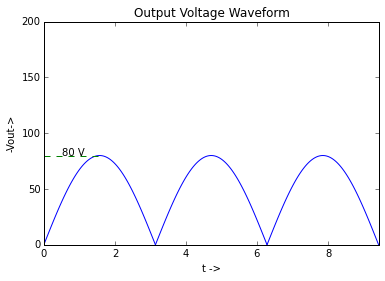## Example 5.14 , Page Number 165¶

In :
#Variables

Vo = 2                     #Output voltage when Vi < 2 V (in volts)
#Vo1 = Vi                  #Output voltage when Vi > 2 V (in volts)

#Calculation

Vo = 2                     #Output voltage during negative half (in volts)

#Result

print "During positive half , Output voltage Vo = 2 V when Vi < 2 V.\nOutput voltage Vo = Vi when Vi > 2 V."
print "During negative half , Output voltage Vo = 2 V."

During positive half , Output voltage Vo = 2 V when Vi < 2 V.
Output voltage Vo = Vi when Vi > 2 V.
During negative half , Output voltage Vo = 2 V.


## Example 5.15 , Page Number 166¶

In :
import math
import numpy
%matplotlib inline
from matplotlib.pyplot import plot,title,xlabel,ylabel,ylim,xlim

#Variables

Vi = 10                    #Input voltage (in volts)
V1 = 2.5                   #Voltage (in volts)
Rnet = 3 * 10**3           #Net resistance (in ohm)
R1 = 2.0 * 10**3           #Resistance1 (in ohm)
R2 = 1.0 * 10**3           #Resistance2 (in ohm)

#Calculation

I = (Vi - V1)/Rnet         #Current (in Ampere)
Vo = I * (R2) + 2.5        #Output voltage positive half (in volts)
Voneg = -Vi                #Output voltage negative half (in volts)

#Result

print "During positive half , Output voltage : ",Vo,"V."
print "During negative half , Output voltage : ",Voneg,"V."

#Graph

x = numpy.linspace(0,9,100)
x1 = numpy.linspace(0,3,100)
x2 = numpy.linspace(3,6,100)
x3 = numpy.linspace(6,9,100)
y1 = numpy.linspace(-10,5,100)
plot(x,0+x-x,'k')
plot(x1,-10+x1-x1,'--',color='g')
plot(x1,5-x1+x1,'b')
plot(x2,-10+x2-x2,'b')
plot(x3,5+x3-x3,'b')
plot(3+y1-y1,y1,'b')
plot(6+y1-y1,y1,'b')
title("Output Voltage Waveform")
xlabel("t ->")
ylabel("-Vout->")
xlim(0,9)
ylim(-12,10)

During positive half , Output voltage :  5.0 V.
During negative half , Output voltage :  -10 V.

Out:
(-12, 10)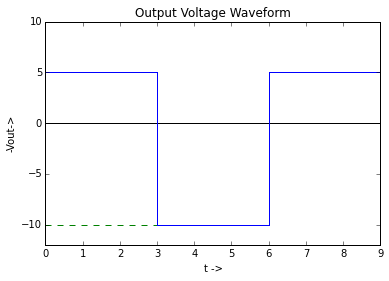## Example 5.16 , Page Number 166¶

In :
import math
import numpy
%matplotlib inline
from matplotlib.pyplot import plot,title,xlabel,ylabel,ylim,xlim

#Variables

V1 = 12              #Voltage1 (in volts)
V2 = 8               #Voltage2 (in volts)

#Calculation

Vopos = V1           #Peak Output voltage during positive half (in volts)
Voneg = -V2          #Peak Output voltage during negative half (in volts)

#Result

print "During positive half , peak voltage : ",Vopos,"V.\nDuring negative half , peak voltage : ",Voneg,"V."

#Graph

x = numpy.linspace(0,24,100)
x1 = numpy.linspace(0,2,100)
x2 = numpy.linspace(2,6,100)
x3 = numpy.linspace(6,8,100)
x4 = numpy.linspace(8,8+8.0/6,100)
x5 = numpy.linspace(8+8.0/6,12+8.0/6,100)
x6 = numpy.linspace(12+8.0/6,12+2*8.0/6,100)
x7 = numpy.linspace(12+2*8.0/6,14+2*8.0/6,100)
x8 = numpy.linspace(14+2*8.0/6,18+2*8.0/6,100)
x9 = numpy.linspace(18+2*8.0/6,20+2*8.0/6,100)
x10 = numpy.linspace(0,8+8.0/6,100)
plot(x,0+x-x,'k')
plot(x1,6*x1,'b')
plot(x2,12-x2+x2,'b')
plot(x3,12-6*(x3-6),'b')
plot(x4,-6*(x4-8),'b')
plot(x5,-8+x5-x5,'b')
plot(x6,-8+6*(x6-(12+8.0/6)))
plot(x7,6*(x7-(12+2*8.0/6)),'b')
plot(x8,12-x8+x8,'b')
plot(x9,12-6*(x9-(18+2*8.0/6)),'b')
plot(x10,-8+x10-x10,'--',color='g')
plot(x1,12+x1-x1,'--',color='g')
ylim(-20,15)
xlim(0,20+2*8.0/6)
title("Output Voltage Waveform")
xlabel("t ->")
ylabel("-Vout->")

During positive half , peak voltage :  12 V.
During negative half , peak voltage :  -8 V.

Out:
<matplotlib.text.Text at 0x9864710>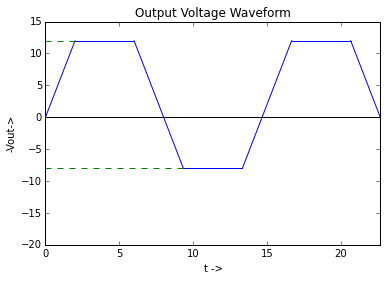## Example 5.17 , Page Number 167¶

In :
import math
import numpy
%matplotlib inline
from matplotlib.pyplot import plot,title,xlabel,ylabel,ylim,xlim

#Variables

Vinmax1 = 25                   #Peak input voltage1 (in volts)
Vinmax2 = 60                   #Peak input voltage2 (in volts)

#Calculation

Voutmax1 = 20                  #Peak output voltage1 (in volts)
Voutmax2 = 10                  #Peak output voltage2 (in volts)

#Result

print "Following is the output wave generated when Vinmax is changed to 60 V."

#Graph

x = numpy.linspace(0,24,100)
x1 = numpy.linspace(0,2,100)
x2 = numpy.linspace(2,6,100)
x3 = numpy.linspace(6,8,100)
x4 = numpy.linspace(8,10,100)
x5 = numpy.linspace(10,14,100)
x6 = numpy.linspace(14,16,100)
x7 = numpy.linspace(0,10,100)
plot(x,0+x-x,'k')
plot(x1,10*x1,'b')
plot(x2,20-x2+x2,'b')
plot(x3,20-10*(x3-6),'b')
plot(x4,-10*(x4-8),'b')
plot(x5,-20+x5-x5,'b')
plot(x6,-20+10*(x6-14))
plot(x7,-20+x7-x7,'--',color='g')
xlim(0,16)
ylim(-22,22)
title("Output Voltage Waveform")
xlabel("t ->")
ylabel("-Vout->")

Following is the output wave generated when Vinmax is changed to 60 V.

Out:
<matplotlib.text.Text at 0x9d5c490>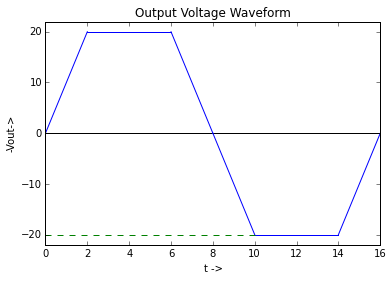## Example 5.18 , Page Number 168¶

In :
import math
import numpy
%matplotlib inline
from matplotlib.pyplot import plot,title,xlabel,ylabel,ylim,xlim,annotate

#Variables

Vinmax = 10.0                   #Peak input voltage (in volts)
R1 = 5 * 10**3                  #Resistance1 (in ohm)
R2 = 5 * 10**3                  #Resistance2 (in ohm)

#Calculation

Vout = Vinmax/(R1 + R2)*R2      #Output voltage (in volts)

#Result

print "Output voltage Vout = Vin/2."
print "Voutmax = ",Vout,'V.'

#Graph

x = numpy.linspace(0,12,100)
x1 = numpy.linspace(0,3,100)
x2 = numpy.linspace(3,6,100)
x3 = numpy.linspace(6,9,100)
y1 = numpy.linspace(-5,0,100)
plot(x,0+x-x,'k')
plot(x1,0-x1+x1,'b')
plot(x2,-5+x2-x2,'b')
plot(x3,0+x3-x3,'b')
plot(3+y1-y1,y1,'b')
plot(6+y1-y1,y1,'b')
plot(9+y1-y1,y1,'b')
plot(x2,-2+x2-x2,'--',color='g')
title("Output Voltage Waveform")
xlabel("t ->")
ylabel("-Vout->")
xlim(0,9)
ylim(-6,5)
annotate('T/2',xy=(4.25,-2))

Output voltage Vout = Vin/2.
Voutmax =  5.0 V.

Out:
<matplotlib.text.Annotation at 0xa9b53f0>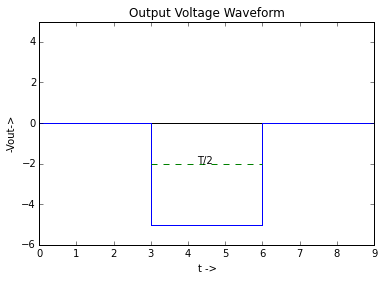## Example 5.19 , Page Number 168¶

In :
import math
import numpy
%matplotlib inline
from matplotlib.pyplot import plot,title,xlabel,ylabel,ylim,xlim,annotate

#Variables

Vinmax = 10                             #Peak input voltage (in volts)
V1 = 5.3                                #Voltage source1 (in volts)
V2 = 7.3                                #Voltage source2 (in volts)
R = 10.0 * 10**3                        #Resistance (in ohm)

#Calculation

Vomax = 6                               #Peak output voltage in positive half (in volts)
Vomin = -8                              #Peak output voltage in negative half (in volts)

#Result

print "OUtput voltage lies in range : -8 V to +6 V."

#Graph

x = numpy.linspace(0,24,100)
x1 = numpy.linspace(0,3,100)
x2 = numpy.linspace(3,5,100)
x3 = numpy.linspace(5,7,100)
x4 = numpy.linspace(7,10,100)
x5 = numpy.linspace(10,14,100)
x6 = numpy.linspace(14,15,100)
x7 = numpy.linspace(15,16,100)
x8 = numpy.linspace(16,20,100)
x9 = numpy.linspace(0,5,100)
x10 = numpy.linspace(0,14,100)
x11 = numpy.linspace(0,15,100)

plot(x,0+x-x,'k')
plot(x1,2*x1,'b')
plot(x2,2*x2,'--',color='b')
plot(x3,10-2*(x3-5),'--',color='b')
plot(x4,6-2*(x4-7),'b')
plot(x5,-2*(x5-10),'b')
plot(x6,-8-2*(x6-14),'--',color='b')
plot(x7,-10+2*(x7-15),'--',color='b')
plot(x8,-8+2*(x8-16),'b')

plot(x1,6-x1+x1,'--',color='g')
plot(x2,6-x2+x2,'k')
plot(x3,6-x3+x3,'k')
plot(x6,-8-x6+x6,'k')
plot(x7,-8-x7+x7,'k')
plot(x9,10+x9-x9,'--',color='g')
plot(x10,-8+x10-x10,'--',color='g')
plot(x11,-10+x11-x11,'--',color='g')

xlim(0,20)
ylim(-12,12)
title("Output Voltage Waveform")
xlabel("t ->")
ylabel("-Vout->")

OUtput voltage lies in range : -8 V to +6 V.

Out:
<matplotlib.text.Text at 0xccf9450>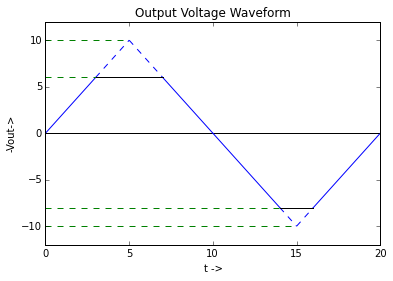## Example 5.20 , Page Number 173¶

In :
import math
import numpy
%matplotlib inline
from matplotlib.pyplot import plot,title,xlabel,ylabel,ylim,xlim

#Variables

Vin = 20                      #Input voltage (in volts)
V1 = 5                        #Battery voltage (in volts)

#Calculation

Voutp = (2*Vin - V1)          #Output voltage in positive half (in volts)
Voutn = 0 - V1                #Output voltage in negative half (in volts)

#Result

print "Output voltage in positive half : ",Voutp,"V."
print "Output voltage in negative half  :",Voutn,"V."

#Graph

x = numpy.linspace(0,9,100)
x1 = numpy.linspace(0,3,100)
x2 = numpy.linspace(3,6,100)
x3 = numpy.linspace(6,9,100)
y1 = numpy.linspace(-5,35,100)
plot(x,0+x-x,'k')
plot(x1,-10+x1-x1,'--',color='g')
plot(x1,35-x1+x1,'b')
plot(x2,-5+x2-x2,'b')
plot(x3,35+x3-x3,'b')
plot(3+y1-y1,y1,'b')
plot(6+y1-y1,y1,'b')
title("Output Voltage Waveform")
xlabel("t ->")
ylabel("-Vout->")
xlim(0,9)
ylim(-10,40)

Output voltage in positive half :  35 V.
Output voltage in negative half  : -5 V.

Out:
(-10, 40)